Examples of Freudenthal-Kantor Triple Systems | OMICS International
Journal of Generalized Lie Theory and Applications

# Examples of Freudenthal-Kantor Triple Systems

Noriaki Kamiya*

Department of Mathematics, University of Aizu, Aizuwakamatsu, 965-8580, Japan

*Corresponding Author:
Norikai kamiya
Department of Mathematics University
of Aizu, Aizuwakamatsu
965-8580, Japan
Tel: +0242-37-2500
E-mail: [email protected]

Received date: January 26, 2014; Accepted date: June 02, 2014; Published date: June 10, 2013

Citation: Kamiya N (2014) Examples of Freudenthal-Kantor triple systems. J Generalized Lie Theory Appl 8:210. doi:10.4172/1736-4337.1000210

Copyright: © 2014 Kamiya N. This is an open-access article distributed under the terms of the Creative Commons Attribution License, which permits unrestricted use, distribution, and reproduction in any medium, provided the original author and source are credited.

Visit for more related articles at Journal of Generalized Lie Theory and Applications

#### Abstract

Symmetry group of Lie algebras and superalgebras constructed from (ϵ,δ) Freudenthal-Kantor triple systems has been studied. Also, the definition and examples of hermitian triple systems is introduced in this note.

#### Keywords

Triple systems; Lie algebras; Symmety group

#### AMS classification

Triple systems; Lie algebras; Symmety group

#### Introduction

It seems that the concept of a triple system (or called a ternary algebra) in nonassociative algebras started from the metasymplectic geometry due to Freudenthal. After a generalization of the concept has been studied by Tits, Koecher, Kantor, Yamaguti, Allison and authors ([1,2] for many earlier references on the subject). Also it is well known the object of investigation of Jordan and Lie algebras with application to symmetric spaces or domains  and to physics [4,5].

Nonassociative algebras are rich in of mathematics, not only for pure algebra differential geometry, but also for representation theory and algebraic geometry. Specialy, the Lie algebras and Jordan algebras plays an important role in many mathematical and physical objects. As a construction of Lie algebras as well as Jordan algebras, we are interested in characterizing the Lie algebras from view point of triple systems [6-9]). These imply that we are considering to structure of the subspase L1 of the five graded Lie (super)algebra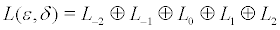satisfying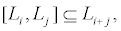associated with an (ε,δ) Freudenthal-Kantor triple system which contains a class of Jordan triple systems related 3 graded Lie algebra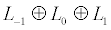. For these consideretions without untilizing properties of root systems or Cartan matrices, we would like to refer to the articles of the present author and earlier references quoted therein [1,2,10,11].

In particular, for an characterizing of Lie algebras, an applying to geometry and physics, we will introduce couple topics about a symmetry of Lie algebras (section 1) and a definition of hermitian triple systems (section 2) in this note, which is a survey and an announcement of new results.

More precisely speaking, first, the symmetry group of Lie algebras and superalgebras constructed from (ε,δ) Freudenthal-Kantor triple systems has been studied. Especially, for a special (ε, ε) Freudenthal- Kantor triple, it is SL(2) group. Secondly, we will give a definition of hermitian * generalized Jordan triple systems and the examples of their tripotents defined by elements c of triple systems satisfying CCC=C.

Symmetry of Lie algebras associated with triple sytems

A triple system V is a vector space over a field F of characteristic ≠ 2 or 3 with a trilinear map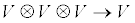. We denote the trilinear product ( or ternary product) by juxtaposition (xyz) ε V(or<xyz>,[xyz],etc).

A well studied triple system is the (ε,δ) Freudenthal-Kantor triple systems ( abbreviated hereafter as to (ε,δ) FKTS) with ε and δ being either +1 or -1 [1,2,9,12].

Since (ε,δ) Freudenthal-Kantor triple systems offer a simple method of constructing Lie algebras (for the case of (δ=+1)) and Lie superalgebras (for the case of δ = -1) with 5-graded structure, it may be of some interest to study its symmetry group in this note. In order to facilitate the discussion, let us briefly sketch its definition.

We introduce two linear mappings L and K:V⊕V →End V by

L (x,y) = xyz, K (x,y)z= - δyzx(1.1)

for δ = +1 or -1 If they satisfy

[L(u,v), L(x,y)] = L(L(u,v)x,y)+ εL(x,L(v,u)y), (1.2)

K(K(u,v)x,y) = L(y,x)K(u,v)- ε K(u,v)L(x,y)(1.3)

for any u,v,x,y ε V and ε =+1we call the triple system to be (ε,δ) FKTS

One consequence of Eqs.(1.2) and (1.3) is the validity of the following important identity (see [Y-O.] Eqs.(2.9) and (2.10))

K(u,v)K(x,y) = ε δL(K(u,v)y,x- εL(K(u,v)x,y)(1.4)

= L(v,K(x,y)u) – δL(u,K(x,y)v) (1.5)

We note that (-1,1)FKTS is said to be a generalized Jordan triple system of second order  in section 2, becaue that is a generalization of concept of Jordan triple systems.

We can then construct a Lie algebra for δ =+1 and a Lie superalgebra for δ =-1 as follows:

Let W be a space of 2 × 1matrices over V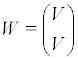and define a tri-linear product:

W ⊕ W ⊕ W→ W by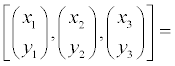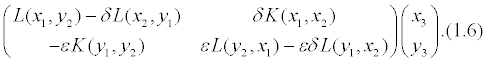Then, it defines a Lie triple system for δ =+1 and an anti-Lie triple system for δ =-1 We then note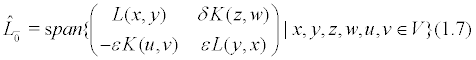is a Lie subalgebra of Mat2(End(V))- where B- for an associative algebra B implies a Lie algebra with bracket; [x,y] = xy-yx We note also then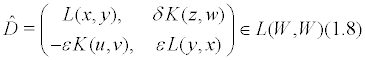is a derivation of the triple system. Setting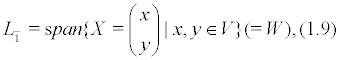then L defined by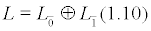gives a Lie algebra for δ =+1 and a Lie superalgebra for δ =-1 where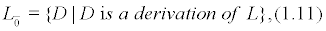i.e., D satisfies

= D[X1,X2,X3]

[DX1,X2,X3]+ [X1, D X2,X3]+ [X1, X2, D X3] (1.12a)

and hence induces also

[D,[X,Y]] = [DX,Y]+[X,DY], (1.2b)

if we define the bracket by

[D1⊕X1,D1⊕X2] = ([D1,D2] + L(X1,X2)) ⊕ (D1X2 - D2X1). (1.13)

where

[D1,D2] = D1,D2 – D2,D1

and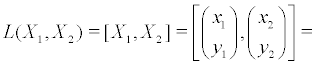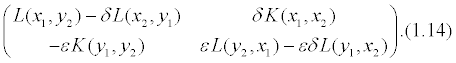Note that the endomorphism L (X,Y) is then an inner derivation of the triple system.

Since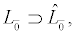we will mainly discuss a subsystem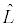of L, given by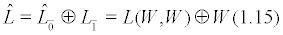rather than the larger L . Then,is 5 -graded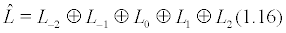where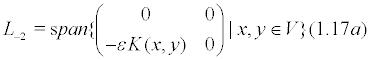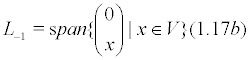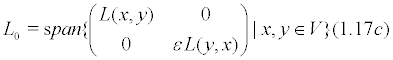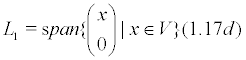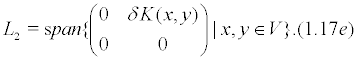Here, we utilized the following Proposition for some of its proof.

Theorem 1: ([K-O.], [K-M-O.]) Let (V, (xyz))) be a (ε,δ) -Freudenthal-Kantor triple system with an endomorphism P such that P2 = - εδId and P(xyz) = (PxPyPz) Then, (V,[xyz)] is a Lie triple system (for δ=1) and anti-Lie triple system (for δ=-1) with respect to the product

[xyz]=(xPyz)- δ(yPxz)+ δ(xPzy)-(yPzx).

In passing, we note that the standard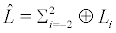is a result of Theorem 1 immediately with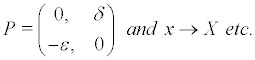Next, we introduce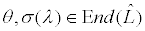for any λ ε F (λ ≠ 0), being non-zero constant by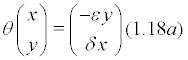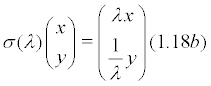in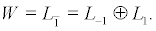We may easily verify that they are automorphism of [W,W,W], i.e, we have for example

θ ([X,Y,Z]) =[ θ X, θ Y, θ Z]for X,Y,Z ε W We then extend their actions to the whole ofin a natural way to show that they will define automorphism of. They moreover satisfy

(i) σ

σ (1)=Id, θ4 = Id(1.19a)

where Id is the identity mapping

(ii)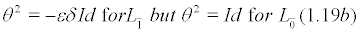(iii) σ(μ)σ(v)= σ(μv) for μ,v ε F, μv ≠ 0

(iv) σ(λ) θ σ(λ) = θ for any λ ε F, λ ≠ 0. (1.19d)

We call the group generated by σ(λ) and θ satisfying these conditions simply as D (ε,δ) due to a lack of better terminology. If the field F contains ω ε F satisfying ω3 = 1 but ω ≠ 1, then a finite sub-group of D (ε,δ) generated by θ and σ (ω ) defines DiC3 group for ε = δ but S3 for ε = -δ, as they have noted already .

Conversly any 5 -graded Lie algebra (or Lie superalgebra) with such automorphism θ and σ(λ) satisfying Eqs.(1.19) lead essentially to a (ε δ) FKTS in L1 with a triple product defined by {x,y,z} = [[x, θ y,]z] for x,y, z ε L1 .

We note that the corresponding local symmetry of D (ε,δ) yields a derivation of, given by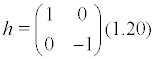which satisfies

h[X,Y,Z] =[ hX,Y,Z]+[X,hY,Z]+[X,Y,hZ](1.21a)

as well as [h,[X,Y]] = [hX,Y] + [X,hY] (1.21b) for X,Y,Z ε W .

We can find a larger automorphism group of, if we impose some additional conditions. First suppose that K(x,y) is now expresed as

K(x,y) = ε δ L(y,x) - ε L(x,y) (1.22)

for any x,y ε V. We call then the triple system to be a special (ε, δ) FKTS . Moreover for the case of special (ε, ε) FKTS (i.e. ε = δ), the automorphism group of ˆL turns out to be a larger SL(2,F)(=Sp(2,F)) group which contains D(ε,ε) as its subgroup. In this case, the triple system [W,W,W] becomes invariant under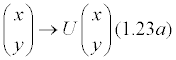for any 2×2 SL(2,F) matrixU,i.e.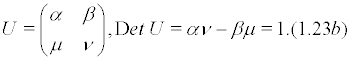Also, the associated Lie algebras or superalgebras are BC1-graded algebra of type C1.

Finally, we consider a ternary system (V, xy, xyz) (V,xy,xyz) where xy and xyz are binary and ternary products, respectively, in the vector space V. Suppose that they satisfy

(1) the triple system (V,xyz) is a (-1,1) FKTS.

(2) The binary algebra (V,xy) is unital and involution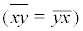with the involutive map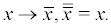(3) The triple product xyz is expressed in terms of the bi-linear products by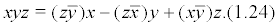We may call the ternary system (V,xy,xyz) to be Allison-ternary algebra or simply A -ternary algebra, since A= (V,xy) is then the structurable algebra [14-16]. This case is of great interest, first because structurable algebras exhbit a triality relation [16,17], and second because we can construct another type of Lie algebras independently of the standard construction of (-1,1) FKTS, which is S4-invariant and of BC1 graded Lie algebra of type B1. The relationship between the Lie algebra constructed in the new way and that given as in Eq.(1.17) is by no means transparent. Note that the group D(-1,1) contains S3 but not S4 symmetry. We may show that if the field F contains the square root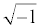of-1, then Eqs.(1.17) can be prolonged to yield the Lie algebra for the structurable algebra.

The Lie algebras constructed as in Eqs.(1.13) and (1.14) is also a BC1-graded Lie algebra of type B1 without assuming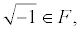(). Also, if F is an algebraically closed field of characteristic zero, then any simple Lie algebra is known to be S4-invariant and can be constructed by some structurable algebra, so that any such Lie algebra is also a BC1- graded Lie algebra of type B1, (as well as of type C1). Of course, the underlying sl(2) symmetry is different for both B1 and C1 cases. Roughly speaking, it seems that these concept are a version of Lie algebras theory corresponding with a Galois group of algebraic numbers theory.

The contents of this section is a cowork with Prof. Okubo, and the details will be discussed in other papers.

Hermitian triple systems

For a geometrical object based on triple systems in this section, first we note that the symmetric bounded domains are a one to one, correspondence to hermite Jordan triple systems, such that a certain trace form is positive definite hermitian. Hence as a generalization of these triple systems, we are interesting to investigate for structure theory of hermitian generalized Jordan triple systems, in particular, the case of hermitian generalized Jordan triple systems of second order (i.e. hermitian (-1,1) -Freudenthal-Kantor triple system ).

We shall concerned with algebras and triple systems which are infinite over a complex number field, unless otherwise specified in this section.

Definition: A triple system V is said to be a *-generalized Jordan triple system of second order if relations (0)--(iv) satisfy;

0) V is a Banach space,

ii) K (<abc>,d)+K(c,<abd>)+K(a,K(c,d)b)= 0,

where L<(a,b)c = <abc> and K(a,b)c =<acb>-<bca>,

iii) <xyz>is C-linear operator on x,z and C-anti-linear operator on y,

iv) <abc> continuous with respect to a norm ║║that is, there exists K>0 such that

║<xxx>║≤K║x║3 for all x ε V.

Furthermore a *-generalized Jordan triple system of second order is said to be hermitian if it satisfies the following condition,

v) all operator L(x,y) is a positive hermitian operator with a hermitian metrix

(x,y) = trace L(x,y),(2.1)

that is, (L(x,y)z,w) = (z,L*(x,y)w), and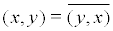. In particular, if a triple system V satisfies the condition (o),(i),(iii),(iv) and (v), then it is said to be a hermitain *-generalized Jordan triple system.

Furthermore, as a generalization of the generalized Jordan triple system of second order, it is said to be a . (ε,δ) -Freudenthal-Kantor triple system if the following relations satisfy;

i) ' [L(a,b),L(c,d)] = L(<abc>,d) εL(c,<bad>)

ii) 'K(<abc>,d)+K(c,<abd>) + δK(a,K(c,d)b) =0,

where K(a,b)c = <abc> -δ<bca>, ε= ±1, δ = ±1.

We note that these identities (i)' and (ii)' are equivalent to the identities (1.2) and (1.3) in section 1 [2,7].

Example 1: Let V be a J*-triple (for the definition, to Loos . Then V is a hermitian *-generalized Jordan triple system of second order, because they satisfy the condition (i) and a special case of (ii) , that is,

K (x,y)z = <xyz> - <yzx>≡0

(identicallly zero).

Example 2: Let T*n,n be the space of diagonal matrix of n×n with element of the complex number. Then T*n,n is a *-generalized Jordan triple system of second order, with respect to the product and the norm ║║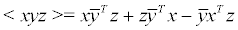for all x,y,z ε T*n,n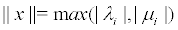where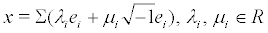, λii∈R (real number) and ei are matrix unit element of T*n,n and xT is the transpose of x.

Next let V be a *-generalized Jordan triple system. Then we may define the notation of a tripotent and a bitripotent as follows.

Definition: It is said to be a tripotent of V if

<ccc> = c,c ε V(2.2).

Definition: It is said to be a strongly bitripotent of V if a pair (c1,c2) of

<c1c1c2> = -1/2c2, < c1c1c2> = -1/2c1, and other porducts are zero.

Definition: It is said to be a bitripotent of V if a pair 1 2 (c ,c ) of the tripotens satisfy the relations

<c1c1c2> = αc2, <c2c2c1> = αc1 ,

< c1c2c1> =βc2,< c2c1c2>=βc1,

<c2c1c1> = γc2, <c1c2c2> = γc1,

and other products are zero, where

α2+ β22 ≠ 0,α,β,γ ε R (real number).

For *-generalized Jordan triple system V, we can define a norm ║║as follows;

║x║= max |λi|,ifx = Σ λiei ε V,

where ei are tripotents or bitripotents. We note that

║x║ ≥ 0and ║x+y║≤║x║+║y║.

Example 3: (a generalization of Example 2.2) Let D*n,k be the set of all n×k matrices with operation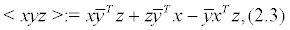where xT and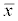mean transpose and conjugation of x respectiverly.

Then D*nk is a hermitian *-generalized Jordan triple system of second order. In fact, the conditions (i),(ii) and (iii) are evident, by straightfoward calculations.

For (v) considering, we have (x,y): = tr (x,y) linear on x and anti linear on y by the condition (iii). Thus we may get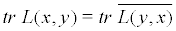as follows.

From the relations;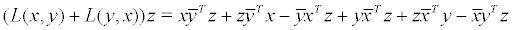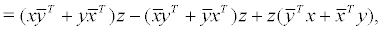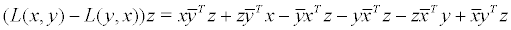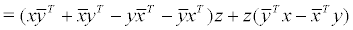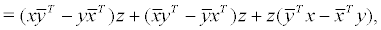it follows that L(x,y)+L(y,x), and L(y,x)-L(y,x) have are a real trace form and an imagenary trace form respectively. Thus we have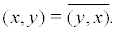To prove positive definite, we consider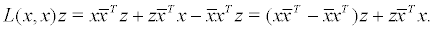Since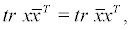we get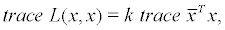this means that the trace from (x,y) is positive definite. It is enough to show the condition (iv). By means of result of the property of matrix, we can write

x=Σμiei, ║x║ = max |μi|,

ei are tripotents or bitripotens if ei is the unit element of matrix.

Moreprecisely speaking, we have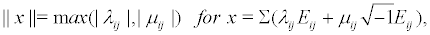where the notations are denoted by λijij ε R (real number field) and also Eij means that (i,j) element is 1 and othere element is zero. Eij and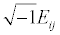are tipotents, i.e., <EijEijEij> = Eij , and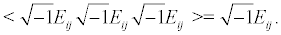Furthermore, we note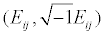are bitripotents.

Then we have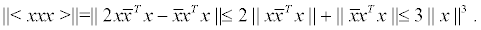These show that D* n,k is a hermilian *- generalized Jordan triple system of second order, that is, a hermitian (-1,1)-FKTS.

Examples 4: Let S*2n,k be the set of all 2n ×k matrices with operation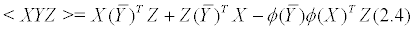where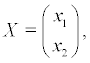xi is an n×k- matrix, and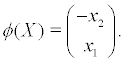Then S*2n,k is a hermitian *-generalized Jordan triple system of second order.

In fact, the elements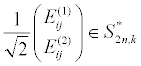are tripotents.

For any element X of S2n,k,we may represent as follows;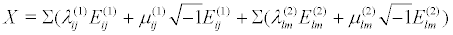and the norm is defined by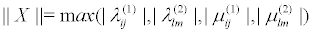where Eij (1) is the matrix unit of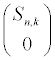and Elm(2) Elm(2) is the matrix unit of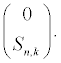By straightforward calculations as well as Example 2.3, we can show that S*2n,k is a hermitian *-generalized Jordan triple system of second order.

Example 4: Let A* kn ⊕ A* nl A* kn be the set fo all pairswhere x1 is a k×n matrix and x2 is a

n × l matrix with operation given by foumula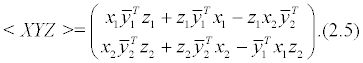Then A*kn ⊕ A* nl is a hermitian *-generalized Jordan triple system of second order.

In fact, for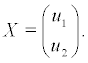we have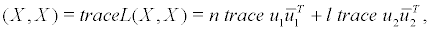hence it follows that the trace form ( , ) is positive definite

For any element of A* kn ⊕ A* nl , we may represent as follows;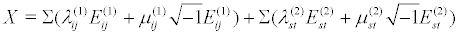and (Eij (1), Ejt (2)) ε A* kn ⊕ A* nl is a tripotent.

Furthermore, the norm is defined by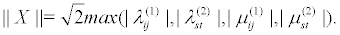Example 6: Let C*nn ⊕ A*nl be the set of all pairswhere x1 is a skew-symmetric n×n matrix and x2 is a column,i.e.,is a n ×1 matrix, with operation given by formula,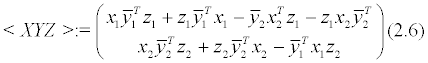Then C*nn ⊕ A*nl is a hermitian *-generalized Jordan triple system of second order.

In fact, we put cij:= the matrix of (i,j) -element is 1,(j,i) -element is -1 and other element is zero (1≤ i ≤ n), further more ek := (0- - - ,1, - - - 0)T,(k th element is 1). Then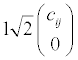are tripotents and also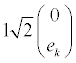are tripotents but these are not bitripotents. For any element of C*nn ⊕ A*nl, we may represent as follow;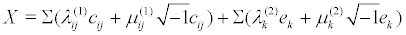and the norm is defined by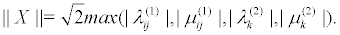In the end of this section, we note a Peirce decomposition as follows ( for the case of δ = 1).

Theorem 1: For δ =±1 and hermitian (-1, δ) Freudenthal-Kantor triple system V with a tripotent C s.t. <ccc> = c we have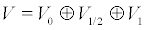where denoted by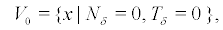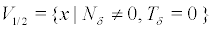,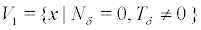and also defined by R(x,y)z=<zxy>, Nδ = L (c,c) – δR(c,c) and Tδ = (1+δ)L(c,c)-R(c,c)+Id.,

This section is a cowork with Dr M.Sato with an application to physics and the details will be considered in future paper .

#### Concluding Remark

In this section, we shall briefy describe a correspondence with the triple systems and the Lie algebras or superalgebras of simple classical type associated with their triple systems [1,2,7,10,11].

Let V be the matrix set of Mat(m,n;F) and the triple product is defined by

<xyz> = xyTz+ δ (zyTx-μzxTy) x,y,z ε V.(*)

Then there exists 4 cases of (-1, δ)-Freudentahl-Kantor triple systems with μ=0 or 1. and δ =±1.

The standard embeding Lie algebras or superalgebras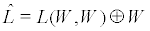, where W = V ⊕ V (cf. section 1) associated with the triple product (*) are appeared by 4 types as follows;

(i) 5 graded Lie algebra (δ =1,μ =1)

Bm+1 = so(2m+2l+1) (n= 2l+1), Dm+1 = so(2m+2l) (n=2l).

(ii) 3 graded Lie algebra (δ =1,μ =0)

An+m-1 =sl(n+m).

(iii) 5 graded Lie superalgebra (δ =-1,μ =1)

. . .B(l,m) =osp(2l+1|2m) (n =2l+1), D(l,m) = osp(2l|2m) (n=2l).

In particular, if m=1, then the triple product

<xyz> = <x,y> z-<z,y> x+<z,x> y

is a (-1,-1)-Freudentahl-Kantor triple system satisfying K(x,z)=<xyz>+<zyx>=2<x,z>y, and so dim{(x,z}span =1 (called a balanced triple system), where <x,y> = x1y1+. . . +xnyn.

(iv) 3 graded Lie superalgebra (δ=-1,μ=0)

A(m-1,n-1) = sl(m|n) (m≠n), A(m-1|m-1) = psl (m|m) (m= n).

#### References

Select your language of interest to view the total content in your interested language

### Article Usage

• Total views: 12262
• [From(publication date):
December-2014 - Nov 23, 2019]
• Breakdown by view type
• HTML page views : 8368Can't read the image? click here to refresh